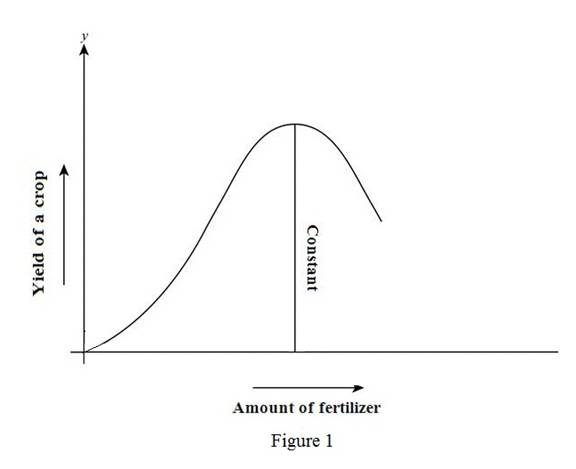# The graph of the yield of a crop as a function of the amount of fertilizer used.### Single Variable Calculus: Concepts...

4th Edition
James Stewart
Publisher: Cengage Learning
ISBN: 9781337687805### Single Variable Calculus: Concepts...

4th Edition
James Stewart
Publisher: Cengage Learning
ISBN: 9781337687805

#### Solutions

Chapter 1, Problem 4RE
To determine

## To sketch: The graph of the yield of a crop as a function of the amount of fertilizer used.

Expert Solution

### Explanation of Solution

Let the amount of fertilizer used be represented in the x-axis and the yield of a crop be represented in the y-axis.

If the amount of fertilizer used is less, then the yield of the crop will also be less in quantity.

Adding more fertilizer will increase the quantity of the crop and it remains constant by adding some more fertilizer.

If the amount of fertilizer is increased even more, then the yield will be decreased.

The rough graph of the yield of a crop as a function of the amount of fertilizer used is shown below in Figure 1.From Figure1, it is observed that the yield of the crop will remain constant for a certain amount of fertilizer.

### Have a homework question?

Subscribe to bartleby learn! Ask subject matter experts 30 homework questions each month. Plus, you’ll have access to millions of step-by-step textbook answers!